# opticspy has a set of Zernike Polynomials Method¶

#### 7. Other helpful methods to use Zernike Polynomials and specific help manual¶

In :
%matplotlib inline
import opticspy


### Generate arbitrary combination Z1 to Z37 Zernike Polynomials surface¶

In :
Z = opticspy.zernike.Coefficient(Z11=1)
Z.zernikesurface()

Z11 =  1 Z40 Primary Spherical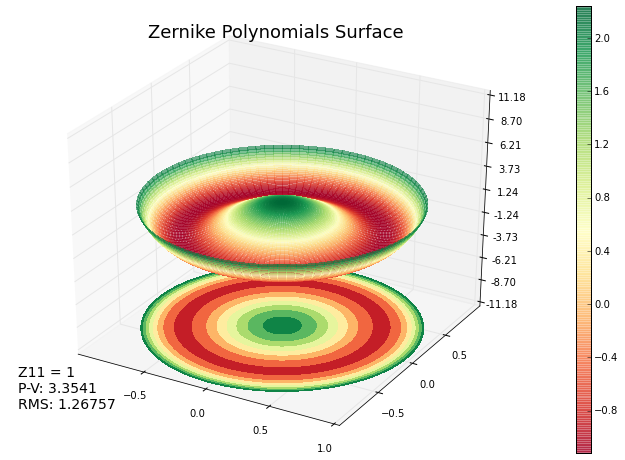In :
Z = opticspy.zernike.Coefficient(Z8=1)
Z.zernikesurface()

Z8 =  1 Z31 Primary x Coma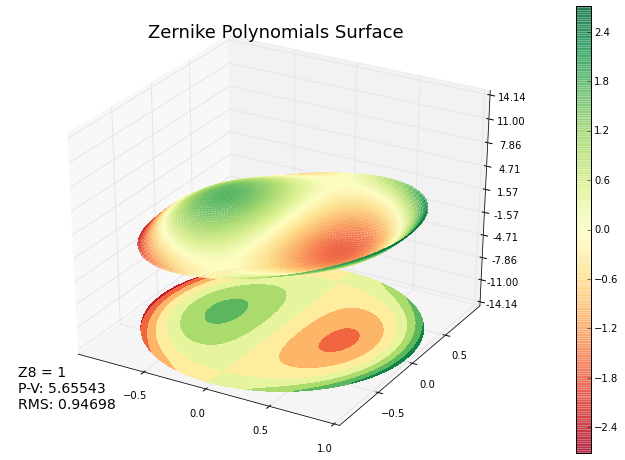In :
Z = opticspy.zernike.Coefficient(Z2=0.1,Z11=0.2,Z15=0.3,Z32=0.1)
Z.zernikesurface()

Z2 =  0.1 Z11 x Tilt
Z11 =  0.2 Z40 Primary Spherical
Z15 =  0.3 Z44 y Tetrafoil
Z32 =  0.1 Z73 Tertiary x Trefoil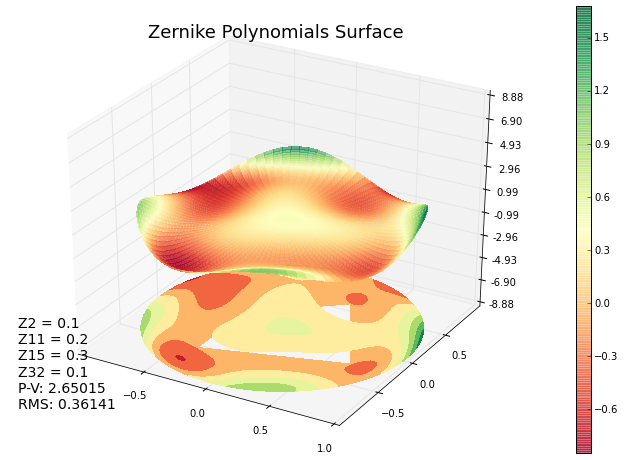### Generate Z1 to Z37 Zernike Polynomials surface 2D map(heatmap)¶

In :
Z = opticspy.zernike.Coefficient(Z11=1)
Z.zernikemap()

Z11 =  1 Z40 Primary Spherical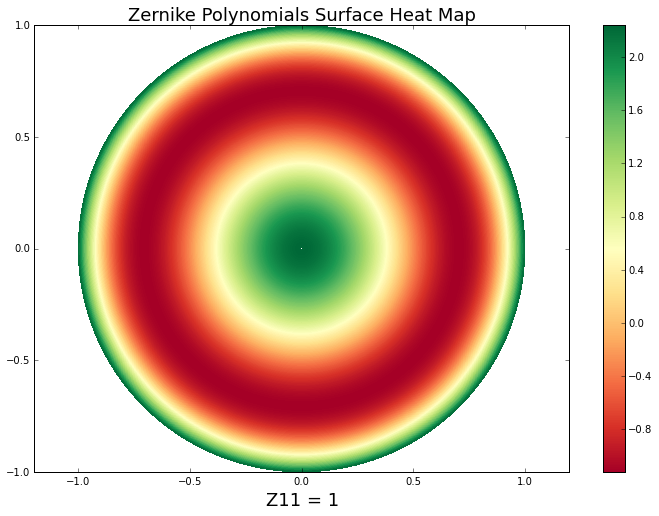In :
Z = opticspy.zernike.Coefficient(Z8=1)
Z.zernikemap()

Z8 =  1 Z31 Primary x Coma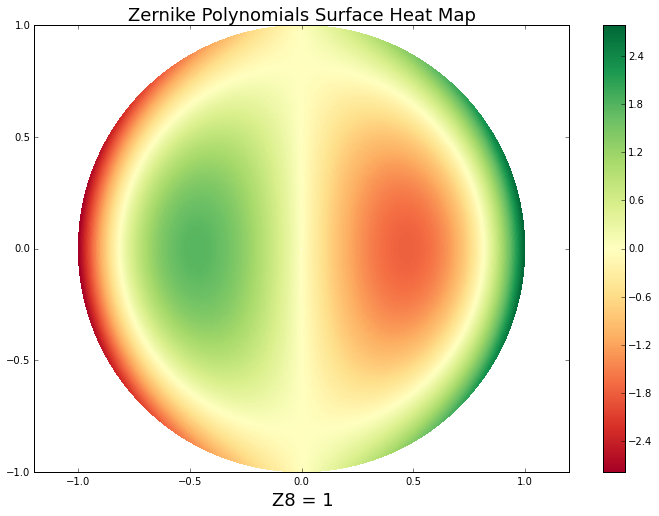In :
Z = opticspy.zernike.Coefficient(Z2=0.1,Z11=0.2,Z15=0.3,Z32=0.1)
Z.zernikemap()

Z2 =  0.1 Z11 x Tilt
Z11 =  0.2 Z40 Primary Spherical
Z15 =  0.3 Z44 y Tetrafoil
Z32 =  0.1 Z73 Tertiary x Trefoil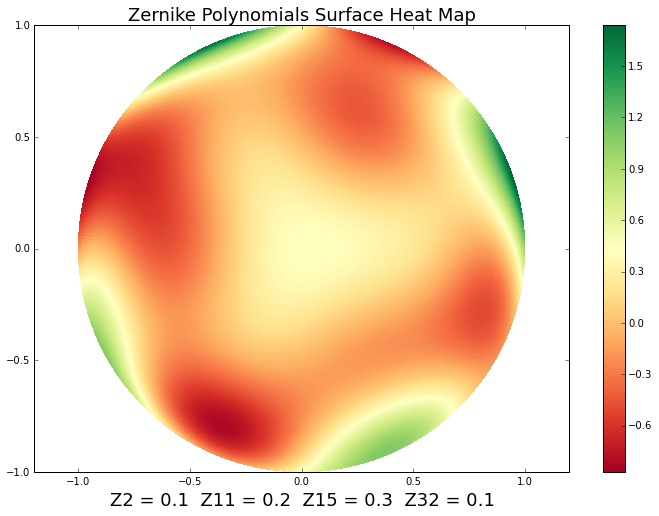### Generate arbitrary combination Z1 to Z37 Zernike Polynomials 1D cutoff through x and y axis of a 3D Zernike Polynomials surface figure¶

In :
Z = opticspy.zernike.Coefficient(Z11=1)
Z.zernikeline()